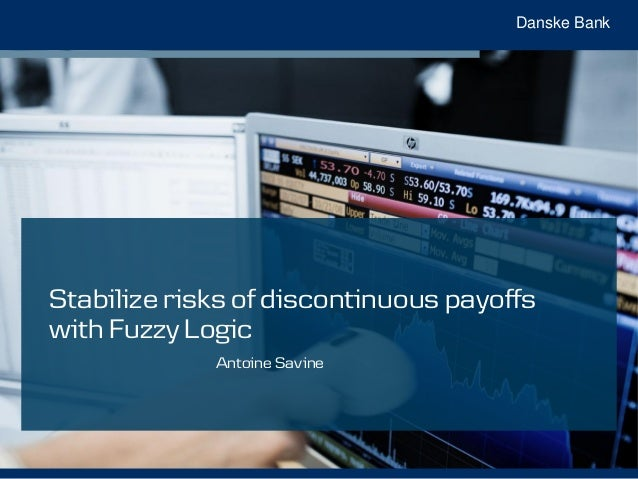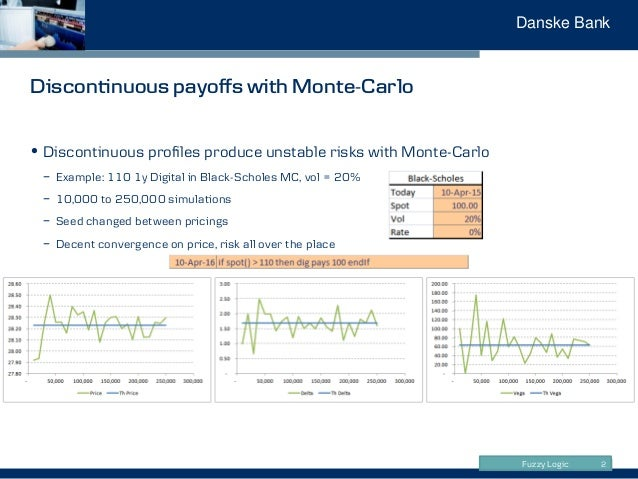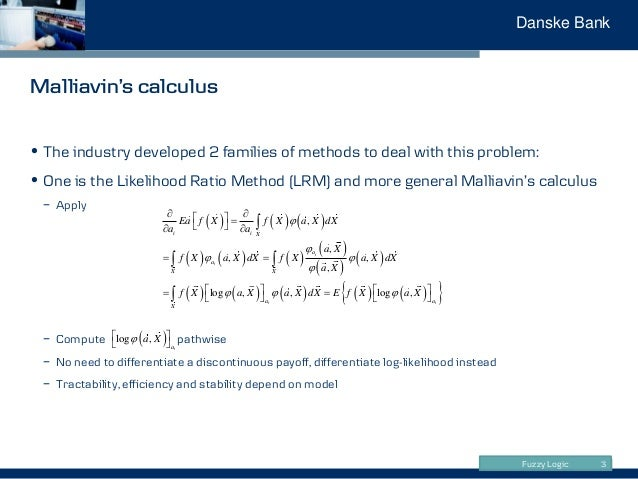Successfully reported this slideshow.Upcoming SlideShare
×

ofUpcoming SlideShare
Next

1 Like

Share

# Stabilise risks of discontinuous payoffs with Fuzzy Logic by Antoine Savine

Antoine Savine's Global Derivatives 2016 Talk
We demonstrate that smoothing, a technique derivatives traders use to stabilise the risk management of discontinuous exotics, is a particular use of Fuzzy Logic.
This realisation leads to a general, automated smoothing algorithm.
The algorithm is extensively explained and its implementation in C++ is provided as a part of our book with Wiley (2018):
Modern Computational Finance: Scripting for Derivatives and xVA

See all

See all

### Stabilise risks of discontinuous payoffs with Fuzzy Logic by Antoine Savine

1. 1. Danske Bank Stabilize risks of discontinuous payoffs with Fuzzy Logic Antoine Savine
2. 2. Fuzzy Logic Danske Bank 2 Discontinuous payoffs with Monte-Carlo • Discontinuous profiles produce unstable risks with Monte-Carlo − Example: 110 1y Digital in Black-Scholes MC, vol = 20% − 10,000 to 250,000 simulations − Seed changed between pricings − Decent convergence on price, risk all over the place
3. 3. Fuzzy Logic Danske Bank Malliavin’s calculus 3 • The industry developed 2 families of methods to deal with this problem: • One is the Likelihood Ratio Method (LRM) and more general Malliavin’s calculus − Apply − Compute pathwise − No need to differentiate a discontinuous payoff, differentiate log-likelihood instead − Tractability, efficiency and stability depend on model                              , , , , , log , , log , i i i i i i X a a X X a a X Ea f X f X a X dX a a a X f X a X dX f X a X dX a X f X a X a X dX E f X a X                                log , ia a X   
4. 4. Fuzzy Logic Danske Bank Payoff smoothing 4 • Payoff smoothing is considerably simpler (and surprinsingly effective): Replace discontinuous payoffs by ”close” continuous ones − Digital  Call spread − Barrier  Smooth barrier • Benefit: work on payoffs only, no work required on models • Problem: to identify (and smooth) all discontinuities we need to know exactly how the payoff is calculated • Today, this is a manual process: Traders rewrite payoffs one by one using a ”call-spread” or ”smooth” function: smooth(x,p,n,e) = 0 e/2-e/2 n p x
5. 5. Fuzzy Logic Danske Bank Smoothing a digital 5 • Digital  Call Spread • Dig =  CS = finite difference continuous approximation • Alt. interpretation: spread the notional evenly across strikes between (K-e/2,K+e/2) C K   
6. 6. Fuzzy Logic Danske Bank Smoothing a barrier 6 • Spread notional across barriers in (B-e/2,B+e/2) • Example: 1y 110-120 RKO in Black-Scholes (20%) • Weekly monitoring, barrier shifted by 0.60 std • Smooth barrier: knock-out part of the notional depending on how far we land in (B-e/2,B+e/2) total KO partial KO no KO
7. 7. Fuzzy Logic Danske Bank Smoothing everything 7 • How can we generalize digital/barrier smoothing to any payoff? • We need to identify (and smooth) all discontinuities • Hence, we need to know exactly how the payoff is calculated • We need access to the ”source code” of the payoff • ”Hard coded” payoffs only allow evaluation against scenarios: ”compiled code” • But scripted payoffs provide full information: ”source code”
8. 8. Fuzzy Logic Danske Bank Scripting Languages 8 • Simple ”programming” language optimized for the description of cash-flows • Describe the cash-flows in any transaction: vanillas, exotics, even CVA/KVA • And then evaluate the script with some model • Example: simplified Autocallable − If spot declines 3 years in a row, lose 50% of notional − Otherwise make 10% per annum until spot raises above initial level
9. 9. Fuzzy Logic Danske Bank The many benefits of scripting cash-flows 9 • Run-time pricing and risks of transactions − Users create/edit a transaction by scripting its cash-flows − Then produce value and risk by sending the script to a model • Homogeneous representation of all cash flows in all transactions − Software can manipulate, merge and compress cash-flows across transactions − Crucial for ”derivatives on netting sets” like VAR/CVA/XVA/KVA/RWA • Crucially for us, provide ”source code” cash-flow information to the software − So the software can, among (many) others: − Obviously, evaluate cash-flows in different scenarios − Detect contingency, path-dependence or early exit and select the appropriate model − Optimise the calculation of value and risk sensitivities − Detect (and smooth) discontinuities
10. 10. Fuzzy Logic Danske Bank Identifying discontinuities 10 • In programming, incl. scripting, discontinuities come from control flow − If this then that pattern − Call to discontinuous functions (meaning themselves implement if this then that statements) • Autocallable: − 3 control flows − Unstable risk
11. 11. Fuzzy Logic Danske Bank Manual smoothing 11 • Replace if this then that by smooth • Does the job, but hard to write and read and error prone, even in simple cases
12. 12. Fuzzy Logic Danske Bank Automatic smoothing 12 • Automatically turn − Nice, easy, natural scripts − Into smoothed scripts that produce stable risks • We have access to the script  we can identify control flows • We only need to smooth them all
13. 13. Fuzzy Logic Danske Bank Fuzzy Logic to the rescue 13 • Turns out we don’t need to transform the script at all! • We just need to evaluate differently the conditions and the conditional statements • In classical (sharp) logic, a condition is true or false − Schrodinger’s cat is dead or alive • In fuzzy logic, a condition is true to a degree = degree of truth or DT − Schrodinger’s cat is dead and alive − For instance, it can be 65% alive and 35% dead • Note: DT is not a probability  Financial interpretation: condition applies to DT% of the notional − A barrier is 65% alive means 35% of the notional knocked out, the rest is still going − It does not mean that it is hit with proba 35%, which makes no sense on a given scenario
14. 14. Fuzzy Logic Danske Bank Fuzzy Logic 14 • Invented in the 1960s • Not to smooth financial risks • But to build the first expert systems • Automate expert opinions − Expert: it is dangerous for the engine to go fast and be hot − Programmer: what do you mean by ”fast” and ”hot”? − Expert: say fast = ”>100mph” and hot = ”>50C” Code: if speed > 100 and heat > 50 then danger = true else danger = false endIf Makes no sense: (99.9,90)  safe, (100.1,50.1)  dangerous !!
15. 15. Fuzzy Logic Danske Bank     1 2 1 2 1 2 1 2 1 2or DT C and C DT DT DT C C DT DT DT DT       More Fuzzy Logic 15 • With fuzzy logic: − isFast and isHot are DTs = smooth functions resp. of speed and heat valued in [0,1] − DTs combine like booleans alt. Code: danger = fuzzyAnd( isFast( speed), isHot( heat)) Results make sense: (99.9,90)  50% dangerous, (100.1,50.1)  25% dangerous         1 2 1 2 1 2 1 2 min , or max , DT C and C DT DT DT C C DT DT  
16. 16. Fuzzy Logic Danske Bank Back to payoff smoothing 16 • Digital smoothing = apply fuzzy logic to if spot>strike then pay 1 • Barrier smoothing = apply fuzzy logic to if spot>barrier then alive = 0 • Classical smoothing exactly corresponds to the replacement of sharp logic with fuzzy logic in the evaluation of conditions • Reversely, we can systematically apply fuzzy logic to evaluate all conditions • Fuzzy logic for control flow effectively removes discontinuities • And stabilises risk sensitivities
17. 17. Fuzzy Logic Danske Bank Evaluation of ”if this then that” with fuzzy logic 17 • Evaluation with sharp logic 1. Evaluate condition C 2. If C is true, evaluate statements between then and else or endIf 3. If C is false, evaluate statements between else and endIf if any • Evaluation with fuzzy logic 1. Record state S0 (all variables and products) just before the ”if” statement 2. Evaluate the degree of truth (DT) of the condition 3. Evaluate ”if true” statements 4. Record resulting state S1 5. Restore state to S0 6. Evaluate ”else” statements if any 7. Record resulting state S2 8. Set final state S = DT * S1 + (1-DT) * S2
18. 18. Fuzzy Logic Danske Bank Fuzzy Logic implementation 18 • No change in scripts • Change the evaluation of if this then that statements only • Effectively stabilises risks sensitivities with minimal PV impact • (With proper optimization) just as fast as normal • Easily flick between sharp (pricing) and fuzzy (risk) evaluation
19. 19. Fuzzy Logic Danske Bank Advanced Fuzzy Logic: condition domains 19 • Consider the script • The variable digPays is valued in {0,1} • To apply fuzzy logic / call spread to digPays > 0 makes no sense • Evidently the DT of digPays > 0 is digPays itself • This is easy to correct but we must first compute the domains of all conditions • Fortunately, we have access to the script • We can write code that traverses the script and calculates condition domains • This is not as hard as it sounds
20. 20. Fuzzy Logic Danske Bank Advanced Fuzzy Logic: beware the cat 20 • We are used to conditions being true or false − Schrodinger’s cat is dead or alive − The cat cannot be dead and alive − If the cat is alive then it can’t be dead, etc. • We may write scripts based on such logic, like for instance: • With fuzzy logic, where spot > 100 to a degree and <= 100 to a degree − The logic that underlies the script is not applicable − And the result is a bias in the value by orders of magnitude! − With fuzzy logic, best stick to the decription of cash-flows and refrain from injecting additional ”logic” X has to be overwritten by either 0 or 1 right?
21. 21. Fuzzy Logic Danske Bank Advanced Fuzzy Logic: what epsilon? 21 • Fuzzy logic applies a ”call spread” to conditions: − Too wide  risk of valuation bias − Too narrow  unstable risks − What is a reasonable size? • Typical e for a condition expr > 0 = fraction of the std dev of expr • Alternative = set e such that Proba ( - e/2 < expr < e/2) = target • Solution: use pre-simulations to estimate the first 2 moments of all conditions • And set their epsilon accordingly
•#### ssuser1145da

Jun. 15, 2020

Total views

609

On Slideshare

0

From embeds

0

Number of embeds

2

50

Shares

0